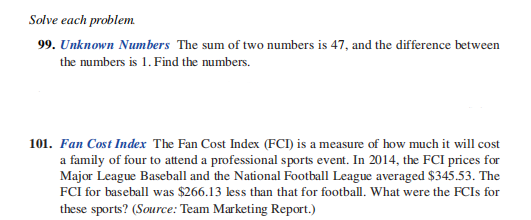### ¿Todavía tienes preguntas de matemáticas?

Pregunte a nuestros tutores expertos
Algebra
Pregunta99. Unknown Numbers The sum of two numbers is $$47$$ , and the difference between the numbers is $$1$$ . Find the numbers.

101. Fan Cost Index The Fan Cost Index (FCI) is a measure of how much it will cost a family of four to attend a professional sports event. In $$2014$$ , the FCI prices for Major League Baseball and the National Football League averaged $$\ 345.53$$ . The FCI for baseball was $$\ 266.13$$ less than that for football. What were the FCIs for these sports? (Source: Team Marketing Report.)

99) x = 24 , y = 23 , 101) $345.53 ,$79.4